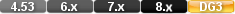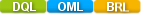Easy to Create, Easy to Change - Easy to use!## Atan2 (arctangent 2)atan2(numerical field,numerical field) atan2(numerical value,numerical value) atam2(numerical field,numerical value) atan2(field,33) atan2(lookup relation field, 3)

The atan2 function calculates the arctangent of Value 1 divided by Value 2

The result is an angle expressed in radians between -p and p.

# Parameters

Numerical values.

Numerical value

# Examples

Example 1

atan2(3,127)

Returns:0.02361766

Example 2

atan2(1, 1)

Returns:0.7854

# atan2 (arctangent 2)

#### Type

Trigonometric Function

#### Purpose

The atan2 function calculates the arctangent of Value 1 divided by Value 2

The result is an angle expressed in radians between -p and p.

Syntax

atan2( NUMERIC VALUE 1, NUMERIC VALUE 2)

A numeric value.

#### Usage

The numeric value in a Trigonometric function can be a constant value (as shown below), a variable, a field value or an expression.

Examples

atan2(3,127)

Returns:0.02361766

atan2(1, 1)

Returns:0.7854